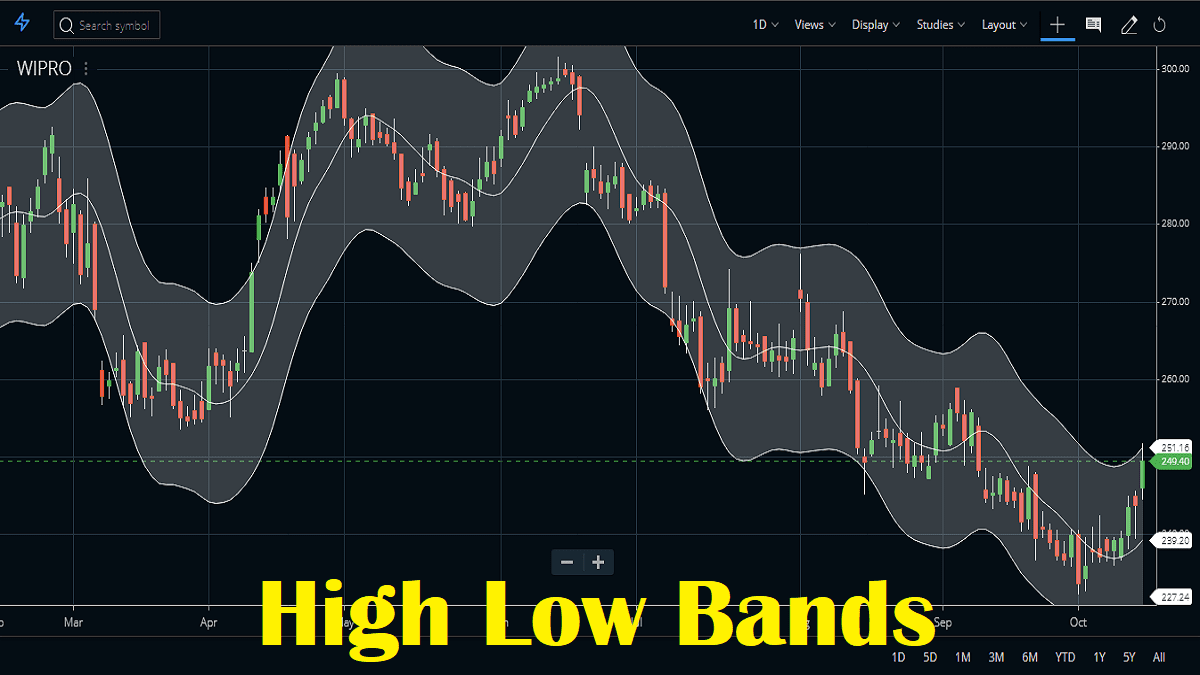Home » Blog » Trading » Technical Indicators » High Low Bands Indicator Trading Strategy, Formula

# High Low Bands Indicator Trading Strategy, FormulaIn High Low Bands Indicator or HLB, there are two bands surrounding the underlying’s price and its generated from the triangular moving averages calculated from the underlying’s price. In this indicator, the triangular moving average is, in turn, shifted up and down by a fixed percentage.

## What is the High Low Bands indicator?

Mainly concept of HLB is based on the triangular moving average. A triangular moving average takes into account, the average price of a specific stock over a particular period of time. In this indicator, triangular moving averages are a bit better than the rest of them because of their ability to generate sharp signals. A triangular moving average is smoother than the others because it is averaged twice. In indicators this is a very simple indicator, but useful for both longs and shorts. This indicator plots the highest high and the lowest low along with the middle point between the two and also an exponential average of the mid-point of the last x periods.

This indicator is also available under the STUDIES section in Zerodha Kite. You can attach it to your chart using the default parameters. Check the example of Reliance Industries price chart in the Kite terminal.

In the High Low Bands indicator, the default period is 10 and the shift percentage is 5. You can change the colour of the upper band, middle band and lower band. The default colours are white for all.

## How to trade using the High Low Bands indicator?

In High Low bands Indicator are some possibilities which can indicate the entry and exit for each trade:

• Buy longs if the upper band is higher than the previous value.
• Or if the midpoint changes the slope from down to up.
• Close longs if lower bands is lower than previous value.
• Or if midpoint changes from the slope from up to down.
• The same procedure for shorts.
• Short sell if the lower band is lower than the previous value.
• Or if the midpoint changes the slope from up to down.
• Close shorts if upper bands are higher than the previous value.
• Or if midpoint changes from the slope from down to up.

In this Indicator, High Low Bands is made up of the triangular moving average of a particular underlying. The triangular moving average for a specific time period is calculated. In this triangular moving average is moved up and down by a specific percentage according to the trader’s preference. This indicator the two bands, thus formed is termed as HLB accordingly. The underlying trades inside this range most of the time. In this underlying breaks out of the band, this generates a signal accordingly. In this indicator, if the underlying pierces the High band on the upside, then we understand it to remain in an uptrend for a certain period of time. Similarly, if the underlying pierces the Low band on the downside, then we understand it to remain in a downtrend for a certain period of time.

## Pros and Cons of High Low Bands Indicator

### Pros of this indicator :

• The High Low Bands Indicator can easily generate signals in trending markets.
• High Low bands are more inclined to price changes.

### Cons of this indicator :

• The High Low Bands Indicator doesn’t seem to work well in choppy or sideways markets.
• The HLB also have much number of waves than any other technical indicator.
• In Zerodha Kite, this indicator plots itself as a band at the end of day time frame or above, in intraday time frames, this is just plotted as a line.
• So this indicator is useless on the intraday time frame.